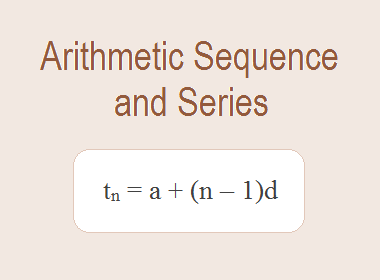Consider the following sequences.

a. 1, 6, 11, 16, ... … …

b. 6, 2, -2, -6, … … … …

c. 3, 4.5, 6, 7.5, … … …

In the first sequence, each term is increased by 5 than the preceding term. In the second sequence, each term is decreased by 4 than the preceding term. Each term in the third sequence is increased by 1.5 than the preceding term. In each of the above sequences, the difference between a term and its preceding term is equal or constant. Such a sequence is said to be an Arithmetic Sequence or Arithmetic Progression.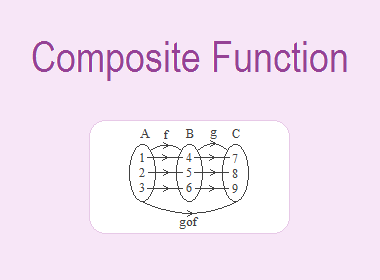Let A = {2, 3, 4}, B = {6, 9, 12} and C = {a, b, c}. If f : A → B and g : B → C are defined by f = {(2, 6), (3, 9), (4, 12)} and g = {(6, a), (9, b), (12, c)},f is the function from A to B such that

2 A, f(2) = 6 B

3 A, f(3) = 9 B

4 A, f(4) = 12 B

Range of f = {6, 9, 12} = domain of g.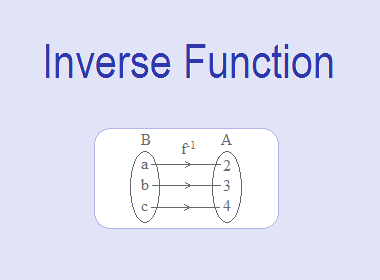Let A = {2, 3, 4}, B = {a, b, c} and the function f : A → B is defined by f = {(2, a), (3, b), (4, c)}.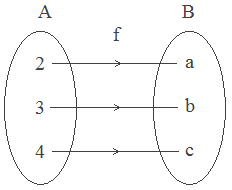Here,

2 A, f(2) = a B

3 A, f(3) = b B

4 A, f(4) = c B

Also domain of f = {2, 3, 4} and range of f = {a, b, c}.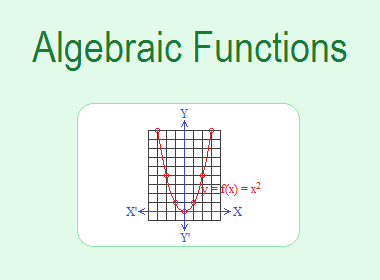Algebraic Functions are those functions which involve the sum or difference of algebraic terms. The following are some different types of algebraic functions.

a. Constant funciton

b. Identity function

c. Linear function

e. Cubic function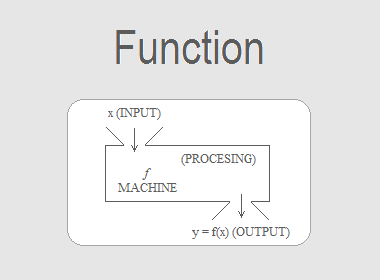Consider two sets A and B. Any non-empty subset R of the cartesian product A × B is called a relation from A to B. A special type of relation associates each element of set A with the one and only element of B. This is indeed a refinement of the concept of relation. Such a refinement is known as a function. We may define a function in the following way:

A function from a set A to a set B is a relation (or rule) which associates each element of A with a unique element of B.

Symbolically, we write

f : A → B

to mean “f is a function from A to B”. Further, an element y of B associated with an element x of A is denoted by f(x). Equivalently, we write y = f(x) which reads ‘y equals f of x’. Here f(x) is known as the image of f at x or the value of f at x.

The letters f, g, h, F, G, H, φ etc. are reserved for denoting functions.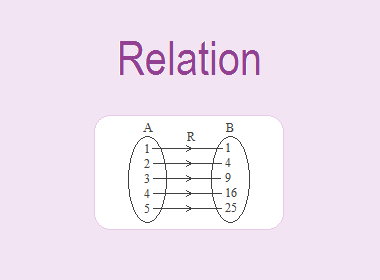If A and B are two non-empty sets then any subset of ordered pairs of a cartesian product A × B is called a relation from set A to set B. A relation is denoted by xRy or simply R, if (x, y) R. A relation from set A to A is called a relation on A.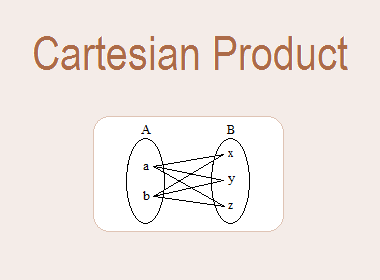If A and B are two non-empty sets then the set of all possible ordered pairs (x, y) such that the first component x is an element of A and the second component y is an element of B is called the Cartesian Product of set A and B. It is denoted by A × B which is read as “A cross B”.

In the set-builder form, we can write

A × B = {(a, b): a A, b B}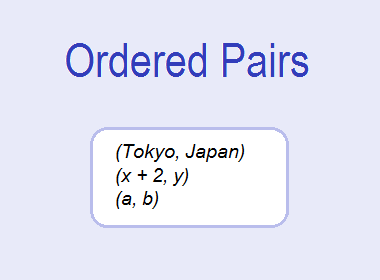An ordered pair is a pair of objects whose components occur in a special (fixed) order. It is written by listing the two components in a specific order, separating them by a comma, and enclosing the pair in parenthesis (small brackets).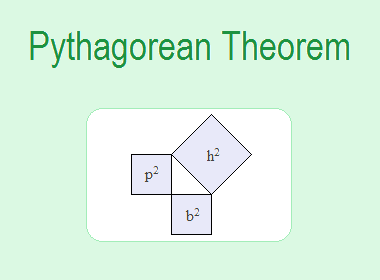Pythagoras (who died about 500 BC) was a Greek philosopher. He was a geometer and a great mystic, who with his followers, seems to have been the first to take mathematics seriously as a study in its own right as opposed to being a collection of formulae for practical calculation. The Pythagoreans are credited with the discovery of the well-known “Pythagoras Theorem” on right-angled triangles.